Optics
Volume 4, Issue 3-1, June 2015, Pages: 43-49

Deformation Measurement Method Using Moiré Fringes at High Scanning Speed Under a Laser Scanning Microscope

Qinghua Wang, Shien Ri, Hiroshi Tsuda, Takashi Tokizaki

Research Institute for Measurement and Analytical Instrumentation, National Institute of Advanced Industrial Science and Technology, Tsukuba, Ibaraki, Japan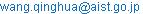(Qinghua Wang)

Qinghua Wang, Shien Ri, Hiroshi Tsuda, Takashi Tokizaki. Deformation Measurement Method Using Moiré Fringes at High Scanning Speed Under a Laser Scanning Microscope. Optics. Special Issue: Optical Techniques for Deformation, Structure and Shape Evaluation. Vol. 4, No. 3-1, 2015, pp. 43-49. doi: 10.11648/j.optics.s.2015040301.20

Received: March 31, 2015; Accepted: April 1, 2015; Published: March 14, 2016

Abstract: A full-field deformation measurement method using moiré fringes generated at high scanning speed under a laser scanning microscope (LSM) was proposed. The formation principle and the deformation measurement principle were presented. Simulation and experiment verifications were performed to show the effectiveness and the accuracy of the proposed fast scanning moiré method, where the scanning line spacing is an integral multiple of the basic scanning line spacing. The moiré spacing, and the deformation measurement sensitivity and accuracy are almost the same as in the traditional LSM scanning moiré method. The fast scanning process allows a high scanning speed and a large view field without reducing the deformation measurement sensitivity. The higher measurement speed makes this method more suitable to be combined with the phase-shifting technique than the traditional LSM scanning moiré method. Besides, the application scope of the LSM moiré method in the field of deformation measurement is extended because more specimen gratings can be used to generate moiré fringes.

Keywords: Deformation Measurement, Strain, High Speed, Fast Scanning Moiré Method, Microscope, Grating

Contents

1. Introduction

Full-field deformation measurement plays an increasingly important role in mechanical properties evaluation, defect detection, instability as well as failure assessment, residual stress measurement  and interfacial bonding strength estimation of multifarious materials and structures. Among various deformation measurement techniques, moiré methods are robust and mature optical methods owing to the non-contact and full-field advantages [2,3]. With the decrease in the sizes of the test objects [4,5], various micron/nano-scale moiré methods have been developed to meet the demands for the micron/nano-scale deformation measurement. The most widely used micron/nano-scale moiré method is the high-power microscope moiré method based on the high-resolution and simple-operation features of high-power microscopes [6,7].

In the last decades, the laser scanning microscope (LSM) moiré method [8-10], the scanning electron microscope (SEM) moiré method [11-14], the atomic force microscope (AFM) moiré method  and the transmission electron microscope (TEM) moiré method  have been investigated and applied to deformation measurement of a variaty of materials and structures. Beacause of the advantages of non-vacuum environment and simple operation , the LSM moiré method have attracted great attention of researchers [8-10,18]. The LSM moiré fringes can be scanning moiré fringes (interference between the specimen grating and the scanning lines), overlapping moiré fringes (superimposed by the specimen gratings before and after deformation) and secondary moiré fringes (interference between the scanning moiré fringes before and after deforamtion) . As the LSM scanning moiré has a greater view field than the LSM overlapping moiré and is easier to be generated than the LSM secondary moiré, the LSM moiré refers to the LSM scanning moiré in this study.

Although the LSM scanning moiré method is simple, the discontinuous maganification of the LSM limits its application and popularization. As the magnifications of the LSM objective lens are usually 5×, 10×, 20×, 50×, 100× and 150×, only some specimen gratings with pitches close to scanning spacing can be used to generate clear moiré fringes. Besides, the scanning moiré image acquisition takes time, making the moiré pattern easier to be affected by the specimen creep.

In this study, we propose to generate LSM moiré fringes at high scanning speed. The application scope of the LSM moiré method is extended and the moiré image acquisition speed is much higher than the traditional scanning moiré method.

2. Moiré Formation at High Scanning Speed

The LSM moiré fringes come from the interference between the the specimen grating and the LSM scaning lines (reference grating). If the pitch of the specimen grating and the spacing of the scanning lines are respectively defined as p and T0 in the direction y, the phase expressions of the specimen grating and the scanning lines will be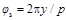and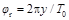, respectively. The direction y is perpendicular to the direction of the scanning lines.

For the generation of the primary (traditional) scanning moiré fringes, there is a small mismatch or misalignment between the scanning lines and the specimen grating, i.e., T0 is close to p. The phase of the primary moiré can be expressed as [12,19]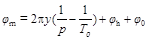(1)

Where φh and φ0 stand for the phase of the higher frequency items (including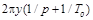,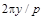) and the initial phase, respectively.

In some cases, it is not easy to meet the condition that the scanning line spacing is close to the specimen grating pitch. Under this circumstance, if the scanning line spacing is close to an integer multiple of the specimen grating pitch, i.e., T(n) is close to np (n is a positive integer), moiré fringes will also emerge as shown in Fig. 1. This case can be realized by scanning the specimen at high scanning speed to make the scanning line spacing greater. The scanning line spacing at high scanning speed can be defined as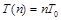(2)

where T0 is the basic scanning line spacing close to the specimen grating pitch (the same meaning as in Eq. (1)). When n=1, T(1)= T0 and the moiré fringes are the same to the primary moiré fringes.

As T(n)/n is close to p, i.e., n/T(n) is close to 1/p, the dominant frequency item of the moiré phase is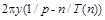. The phase of the moiré fringes at high scanning speed can be represented by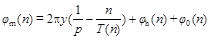(3)

where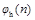and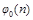denote the phase of the higher frequency items (including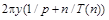,) and the initial phase of the moiré fringes at high scanning speed, respectively.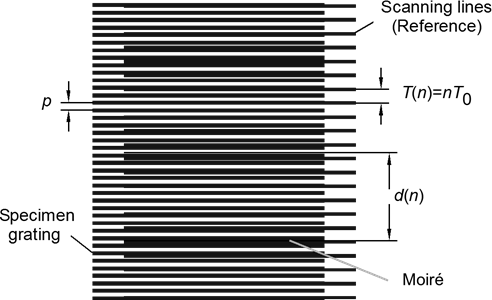Figure 1. Formation principle of moiré fringes at high scanning speed.

3. Principle of Deformation Measurement

As moiré fringes are in approximate periodic distribution, the moiré phase at high scanning speed and the moiré spacing have the following relationship: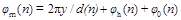(4)

where d(n) is the moiré spacing in the direction y, andandalso indicate the phase of the higher frequency items and the initial phase at high scanning speed, respectively.

By comparing the dominant frequency items of the moiré phase in Eq.(3) and Eq.(4), we can get the relationship between the specimen grating pitch, the scanning line spacing and the moiré spacing as follows: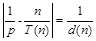(5)

Substituting Eq. (2) into Eq. (5), we can find that the moiré spacing remains unchanged no matter what the value of n is (n=1, 2, 3,…). The following two relationships can be obtained: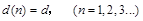(6)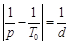(7)

The moiré fringes at high scanning speed are essentially the contours of equal displacement as a function of the basic scanning line spacing T0. If a moiré fringe order is k (integer), the displacement in the direction y at the position of this moiré fringe will be(8)

The strain of the specimen grating (with pitch of p) relative to the basic scanning line (with spacing of T0) is usually given by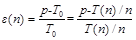(9)

Based on Eq. (7) and Eq. (9), the strain of the specimen grating relative to the basic scanning line can be calculated from the following expressions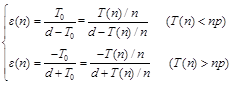(10)

As the moiré spacing is usually much greater than the basic scanning line spacing (d>>T0) in the direction y, Eq. (10) can be simplified to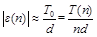(11)

The deformation measurement method from the moiré fringes generated at high scanning speed is called the fast scanning moiré method. The scanning speed is higher than the traditional moiré method. The deformation measurement sensitivity will be discussed in Section 5.

4. Simulation and Experiment Verifications

4.1. Simulation Verification

The phenomenon that the moiré spacing keeps constant regardless of the value of n was verified from simulation and experiments. The deformation measurement accuracy of the fast scanning moiré method was also studied by comparing with the traditional scanning moiré method.

Figure 2 illustrates the simulated (digital sampling) moiré fringes at different scanning resolutions (sampling pitch). A cosine-type parallel grating with pitch of 13 pixels was treated as the specimen grating, as shown in Fig. 2(a). T0=12 pixels was chosen as the basic scanning line spacing. The superposition of the specimen grating and a digital grating with pitch of T0=12 pixels generated the primary moiré pattern, as seen in Fig. 2(b). The digital sampling moiré from the specimen grating at a sampling interval of T(1)=T0=12 pixels also produced the primary moiré pattern (Fig. 2(c)), the same as in Fig. 2(b). As a consequence, the digital sampling moiré was used in comparision and discussion on behalf of superposition moiré and the scanning moiré.

The digital sampling moiré patterns at different sampling intervals T(2)=24, T(3)=36, T(4)=48 are displayed in Fig. 2(d), Fig. 2(e) and Fig. 2(f), respectively. It is clear that the moiré spacing remains unchange no matter what n is (n=1, 2, 3, 4). In other words, the moiré spacing stays the same as long as the scanning line spacing T(n) is n times the basic scanning line spacing T0. It should be mentioned that, the moiré contract decreases with the increase of n, since the gray scales in Figs. 2(a)-(f) are the same ranging from -1 to 1. However, the image contrast can be enhaced to make the moiré fringe clear both in the simulation tests and in the real experiments using image processing technique.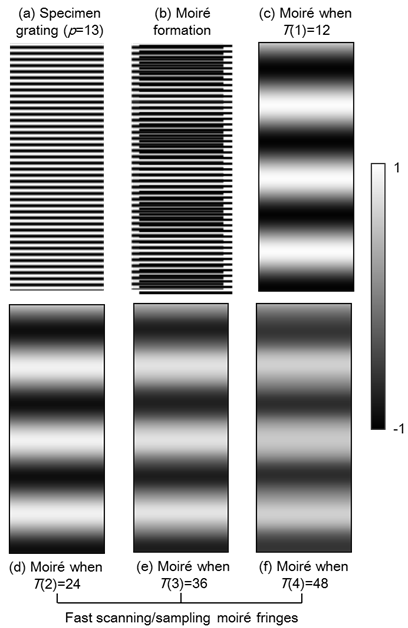Figure 2. Simulation moiré fringes from interference between a specimen grating and reference gratings with different pitches: (a) specimen grating with pitch of 13 pixels, (b) digital sampling moiré from superposition of (a) and a reference grating with pitch of 12 pixels, (c)-(f) simulation moiré from superposition of (a) and reference gratings with pitches of 12 pixels, 24 pixels, 36 pixels and 48 pixels, respectively.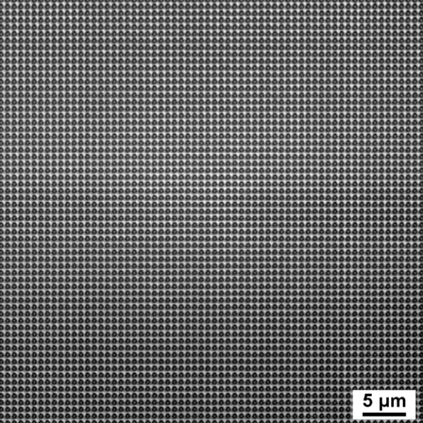Figure 3. Specimen grating fabricated by UV nanoimprint lithography on silicon.

4.2. Experiment Verification

The deformation measurement accuracy was studied from experiments in this section. A cross grating with pitch of around 0.79 μm was first fabricated on a silicon wafer by UV nanoimprint lithography  as presented in Fig. 3.

The silicon wafer with the specimen grating was then put on the sample stage of a laser scanning microscope (Lasertec, OPTELICS HYBRID L3). The scanning line number could be 1024, 512 and 256 in the y direction. When we chose the objective lens with magnification of 20 × and the scanning line number is 512 in the y direction, the scanning line spacing was T(1)=T0=0.726 μm, close to the specimen grating pitch. The primary scanning moiré pattern from the interference between the specimen grating and the scanning line with spacing of 0.726 μm is shown in Fig. 4(a). Under the same objective lens, the scanning line spacing was T(2)=2T0=1.452 μm when the scanning line number was 256. In this case, the fast scanning moiré pattern from the interference between the specimen grating and the scanning line with spacing of 1.452 μm emerged as seen in Fig. 4(b).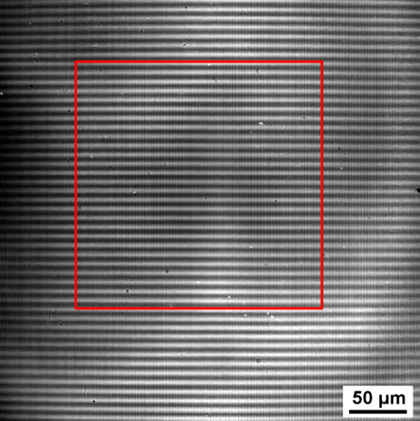(a) The scanning line spacing is T(1)=0.726 μm when the scanning line number is 512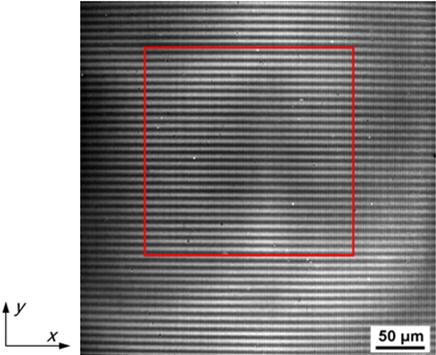(b) The scanning line spacing is T(2)=1.452 μm when the scanning line number is 256

Figure 4. LSM moiré patterns at different scanning resolutions on silicon when the magnification of the objective lens is 20×.

(a) The scanning line spacing is T(1)=0.726μm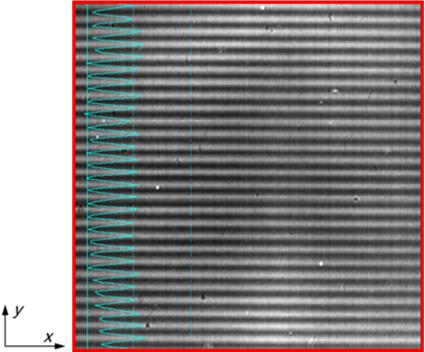(b) The scanning line spacing is T(2)=1.452 μm

Figure 5. Intensity profiles of LSM moiré patterns in the areas (217.7×217.7 μm2) labeled by the red squares in Figs. 4 (a) and (b).

To prevent the influence of the potential scanning distortion on the moiré fringes in the edge region, two same areas labeled by the red squares in Figs. 4(a) and (b) were chosen to be used for deformation measurement. The intensity profiles of the LSM moiré patterns in the labeled areas (217.7×217.7 μm2) in Figs. 4 (a) and (b) were plotted in Figs. 5 (a) and (b), respectively. It can be seen that the intensity profile and the moiré spacing when the scanning line spacing is 0.726 μm are almost the same to those when the scanning line spacing is 1.452 μm. It also confirms the phenomenon that the moiré spacing remain unchanged as long as the scanning line spacing is several times the basic scanning line spacing.

The deformation distributions of the specimen grating relative to the basic scanning lines (T0=0.726 μm) were calculated from the LSM moiré patterns in Figs. 5 (a) and (b) based on Eqs. (8) and (10). The displacement between two moiré fringes was obtained from one-dimensional parabolic interpolation in the software Moiré Analysis. The displacement and the strain distributions calculated from Fig. 5(a) when T(1)=0.726 μm were drawn in Fig. 6, and those calculated from Fig. 5(b) when T(2) =1.452 μm were plotted in Fig. 7.

The average strain in Fig. 6(b) when T(1)=0.726 μm is 0.08992, and the average strain in Fig. 7(b) when T(2)=1.452 μm is 0.08981 relative to the basic scanning lines (T0=0.726 μm). Compared with the result from the traditional (primary) scanning moiré method when T(1)=T0=0.726 μm, the relative error of the strain when T(2)=1.452 μm is only 0.12%, demonstrating the validity of the proposed fast scanning moiré method. The slight differences between the displacement and the strain distributions in Fig. 6 and Fig. 7 are due to vibration and random noise of detector.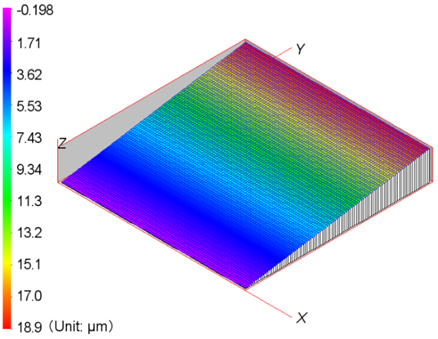(a) Displacement distribution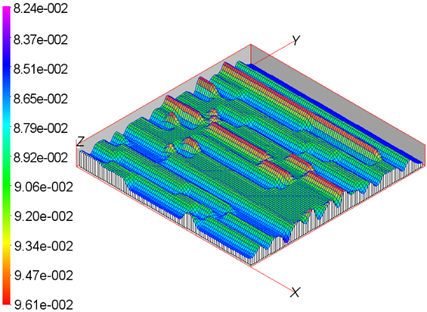(b) Strain distribution

Figure 6. Deformation distributions of the specimen grating relative to the basic scanning lines (T0=0.726 μm) calculated from Figure 5 (a) when T(1)=0.726 μm in an area of 217.7×217.7 μm2.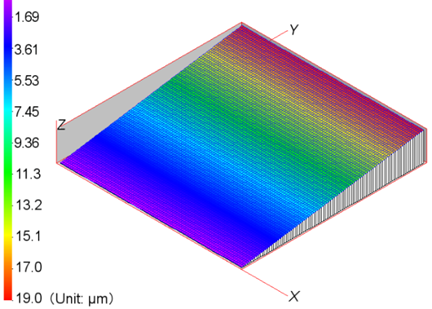(a) Displacement distribution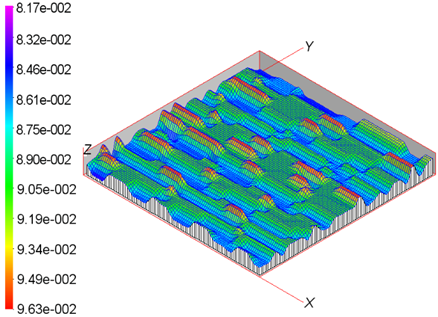(b) Strain distribution

Figure 7. Deformation distributions of the specimen grating relative to the basic scanning lines (T0=0.726 μm) calculated from Figs. 5 (b) when T(2)=1.452 μm in an area of 217.7×217.7 μm2.

5. Discussion

From the simulation and experiment results in Section 4, the moiré spacing remain unchanged if the scanning line spacing is n times the basic scanning line spacing (T0 is close to p), i.e., T(n)=nT0, regardless of the value of n. This phenomenon is different from the case that the moiré spacing changes with the variation of the scanning line spacing in the multiplication moiré method . Experiments show that the high scanning speed only have little effcet on the deformation measurmeent accuracy in the proposed method.

Next, the deformation measurement sensitivity of the proposed method will be analyzed theoretically. From Eq. (8), the displacement sensitivity of the proposed method is T(n)/n per fringe, the same to the displacement sensitivity T0 per fringe in the traditional scanning moiré method. The strain sensitivity is defined as the minimum strain which can be measured, i.e., the strain when there are only two moiré fringes in the full view field, i.e., d=(N(n)-1)T(n), where N(n) is the scanning line number (such as 1024, 512, 256). According to Eq. (11), the strain sensitivity of the proposed method is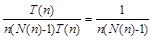. As the full view field can also be expressed as d=(N0-1)T0 (N0 is the basic scanning line number when T0 is close to p), the strain sensitivity can also be calculated from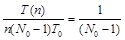based on Eq. (11) and Eq. (2), the same to the strain sensitivity 1/(N0-1) of the traditional moiré method. In other words, both the displacmenet and the strain mearuement sensitivities of the proposed moiré method are the same to those of the traditional scanning moiré method.

The major advantage of the proposed fast scanning moiré method is that the scanning speed is several times higher than the traditional scanning moiré method because the scanning line number is several times less in the same view field. The proposed method is more insusceptible to the specimen creep and more suitable to be combined with the phase-shifting technique to improve the measurement accuracy with high spatial resolution. The high scanning speed can also be realized by using an objective lens with reduced magnification when the scanning line number cannot be adjusted. On this occasion, the view field will be greater than the traditional scanning moiré method, which is another advantage. In addition, as more grating pitches can be used to generate moiré fringes, the application scope of the LSM moiré method for deformation measurement is extended.

6. Conclusions

In this study, a deformation measurement method using moiré fringes generated at high scanning speed, i.e., the fast scanning moiré method was proposed and studied under a laser scanning microscope (LSM). The deformation measurement principle was introduced. The high scanning speed has little effect on the moiré spacing and the deformation measurement sensitivity as well as accuracy. The proposed method has a high image acquisition speed in a large and full view field, making it is more insusceptible to the specimen creep and more suitable to be integrated with the phase-shifting technique. Moreover, gratings with more pitches are usable in the LSM moiré method for the micron/submicron-scale deformation measurement.

Acknowledgement

This work was supported by Cross-ministerial Strategic Innovation Promotion Program - Unit D66 - Innovative Measurement and Analysis for Structural Materials (SIP-IMASM) operated by the Cabinet Office, Japan.

References

1. J. G. Zhu, H. M. Xie, Z. X. Hu, et al., "Residual stress in thermal spray coatings measured by curvature based on 3D digital image correlation technique", Surface & Coatings Technology", Vol. 206(6), 2011, pp. 1396-1402
2. R. Weller and B. Shepard, "Displacement Measurement by Mechanical Interferometry", Proc. Soc. Exp. Stress Analysis, Vol. 6(1), 1948, pp. 35-38
3. B. Han, P. Ifju and D. Post, "Geometric Moiré methods with enhanced sensitivity by optical/digital fringe multiplication", Exp. Mech., Vol. 33 (3), 1993, pp. 195-200
4. M. A. Haque and M. T. A. Saif, "Deformation mechanisms in free-standing nanoscale thin films: A quantitative in situ transmission electron microscope study." Proc. National Academy of Sciences of the United States of America, Vol. 101(17), 2014, pp. 6335-6340
5. Y. J. Li, H. M. Xie, B. Q. Guo, et al., "Fabrication of high-frequency moiré gratings for microscopic deformation measurement using focused ion beam milling", Journal of Micromechanics and Microengineering, Vol. 20(5), 2010, pp. 055037
6. Q. H. Wang, S. Kishimoto, X. F. Jiang and Y. Yamauchi, "Formation of secondary Moiré patterns for characterization of nanoporous alumina structures in multiple domains with different orientations", Nanoscale, Vol. 5, 2013, pp. 2285-2289
7. Q. H. Wang, S. Kishimoto and Y. Yamauchi, "Three-directional structural characterization of hexagonal packed nanoparticles by hexagonal digital moiré method", Opt. Lett., Vol. 37, 2012, pp. 548-550
8. B. Pan, H. M. Xie, S. Kishimoto and Y. Xing, "Experimental study of moiré method in laser scanning confocal microscopy", Rev. Sci. Instrum., Vol. 77, 2006, pp. 043101-043105
9. M. J. Tang, H. M. Xie, Q.H. Wang and J. G. Zhu. "Phase-shifting laser scanning confocal microscopy moiré method and its applications", Meas. Sci. Technol., Vol. 21(5), 2006, pp. 043101-043105
10. H. M. Xie, Q. H. Wang, S. Kishimoto and F. L. Dai, "Characterization of planar periodic structure using inverse laser scanning confocal microscopy moiré method and its application in the structure of butterfly wing", J. Appl. Phys., Vol. 101, 2007, pp. 103511
11. S. Kishimoto, M. Egashira and N. Shinya, "Microcreep Deformation Measurements by a Moiré Method Using Electron-Beam Lithography and Electron-Beam Scan", Opt. Eng., Vol. 32, 1993, pp. 522-526
12. S. Kishimoto, Q. H. Wang, H. M. Xie and Y. Zhao, "Study of the Surface Structure of Butterfly Wings Using the Scanning Electron Microscopic Moiré Method", Appl. Opt., Vol. 46(28), 2007, pp. 7026-7034
13. C. W. Li, Z. W. Liu, H.M. Xie, et al., "Novel 3D SEM Moiré method for micro height measurement’, Optics express, Vol. 21(13), 2013, pp. 15734-15746
14. Q. H. Wang, and S. Kishimoto, "Simultaneous analysis of residual stress and stress intensity factor in a resist after UV-nanoimprint lithography based on electron moiré fringes," J. Micromech. Microeng. Vol. 22, 2012, pp. 105012
15. F. Su, J. Wei and Y. C. Liu, "Removal of AFM moiré measurement errors due to non-linear scan and creep of probe", Nanotechnology, Vol. 16(9), 2005, pp. 1681
16. Y. Androussi, T. Benabbas, and A. Lefebvre. "Moiré-like fringes in transmission electron microscopy images of coherently strained semiconductor islands." Ultramicroscopy, Vol. 93(2), 2002, pp. 161-167
17. R. Rezakhaniha, A. Agianniotis, J. T.C. Schrauwen, A. Griffa, D. Sage and C. V. C. Bouten, "Experimental investigation of collagen waviness and orientation in the arterial adventitia using confocal laser scanning microscopy", Biomech. Model. Mechanobiol. Vol. 11(3-4), 2012, pp. 461-473
18. Q. H. Wang, H. Tsuda, S. Kishimoto, Y. Tanaka, Y. Kagawa. Three moiré techniques under a laser scanning microscope for micron/submicron-scale deformation measurement. Proceedings of the 11th Laser Metrology for Precision Measurement and Inspection in Industry 2014, September 02-05, 2014, Tsukuba, Japan
19. S. Ri, M. Fujigaki, and Y. Morimoto, "Sampling moiré method for accurate small deformation distribution measurement," Experimental Mechanics, Vol. 50, 2010, pp. 501–508
20. Q. H. Wang, S. Kishimoto, Y. Tanaka and Y. Kagawa, "Micro/submicro grating fabrication on metals for deformation measurement based on ultraviolet nanoimprint lithography", Opt. Lasers Eng., Vol. 51 (7), 2013, pp. 944-948
21. Y. J. Li, H. M. Xie, P. W. Chen and Q. M. Zhang, "Theoretical analysis of moiré fringe multiplication under a scanning electron microscope", Meas. Sci. Technol., Vol. 22(2), 2011, pp. 025301

 Contents 1. 2. 3. 4. 4.1. 4.2. 5. 6.
Article ToolsAbstractPDF(3683K)